# Solving Basic Properties of Functions and Equations Made Easy

May 28, 2013 —Comments Off

Recently, we’ve been showcasing some new math features in Wolfram|Alpha, particularly those relevant to primary and secondary school students. Our idea is that when using Wolfram|Alpha, learning math can be a fun experiment. We’d like you to think of Wolfram|Alpha as your own infinitely patient robot, which you can use to explore mathematical ideas, test your knowledge, and generally answer any specific math question you have.

One area we’ve expanded is the basic properties of functions and equations.

The most basic and important class of mathematical functions are the polynomials. A polynomial is a function that involves only multiplication, addition, and whole number powers of its variables. Therefore, anything involving roots, divisions, or other operations on variables is not a polynomial. For example: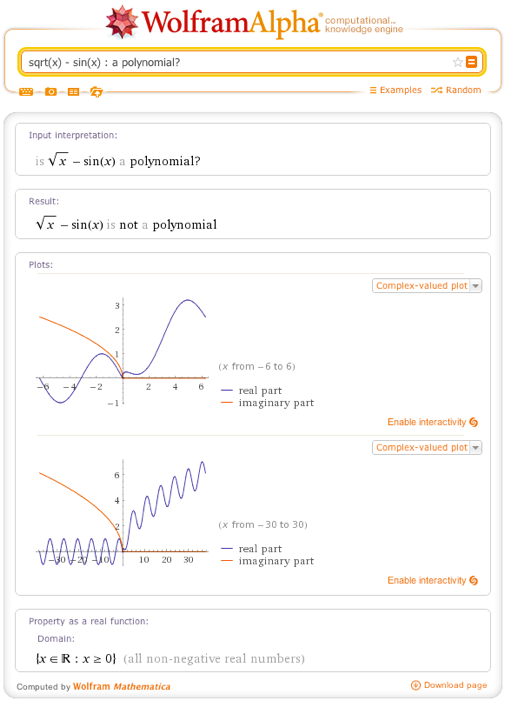A monomial is a polynomial that is just a single term (contains no additions or subtractions). When asked if a complex expression is a monomial/polynomial, Wolfram|Alpha will, if necessary, attempt to simplify the expression to see if it is equal to a monomial/polynomial. If it is, Wolfram|Alpha will show you what it is equal to and, if you click the Step-by-step solution button, explain why this equality is true. For example: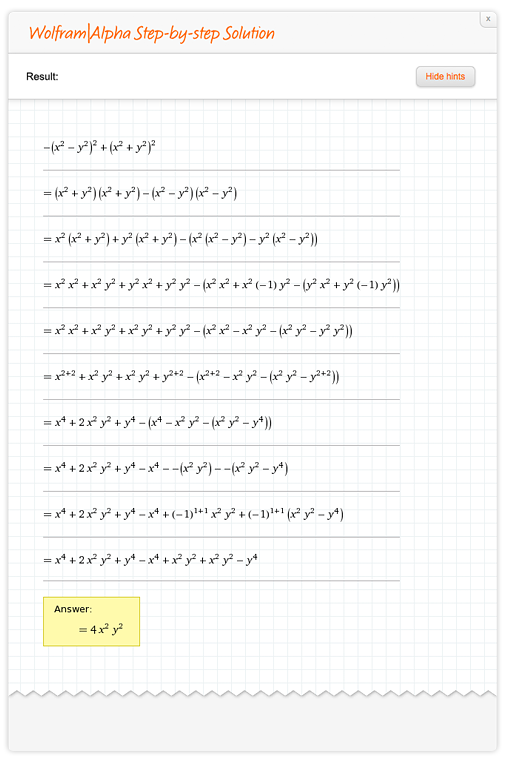You can also ask if a monomial is a term of another polynomial, for example: Is 10x^2 a term of (1+x)^5?

The degree or order of a monomial is the number of variables being multiplied together, including repetitions. (So x2 and x*y are both degree 2, or quadratic, monomials.) The degree of a polynomial is the maximum degree of its monomial terms. Higher-degree polynomials are used to represent more complex models, because there are more degrees of freedom (parameters) to adjust. A first-degree polynomial represents a linear model, a second-degree polynomial yields a parabola, and so on.

If you ask about the degree of a polynomial, Wolfram|Alpha will tell you the degree and the highest-degree terms and their coefficients. If necessary, it will also expand the expression and rearrange the terms, so that you can easily see why the degree is what it is. If you click the Step-by-step solution button, it will also show you the steps involved in this expansion.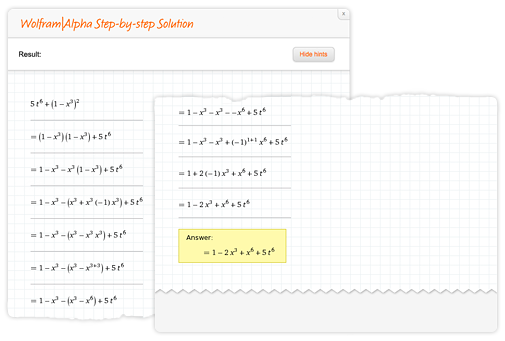Rational functions (ratios of polynomials) also have a notion of degree. When expressed in lowest terms (canceling out common factors), the degree of a rational function is the maximum of the degree of the numerator and of the denominator.Here’s a mathematical riddle: How is 5 even?

In the usual sense of the words, we all know that the number 5 is odd, not even: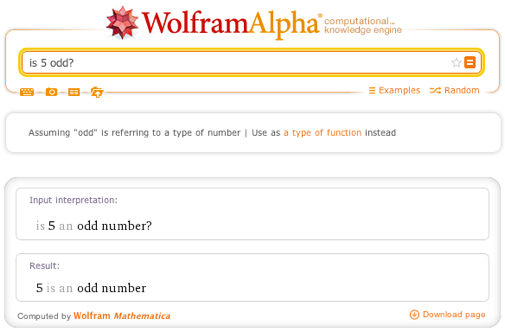You’re probably thinking of sneaky ways to interpret the word even, but in this case it helps to realize that 5 also has different interpretations. Besides a numeral and a number, it could also mean the function 5, that is, the function f such that for every value of x, f(x) = 5.

Just like whole numbers, functions can be odd or even (although unlike whole numbers, most functions are neither). When referring to functions, odd means that, for all x, f(-x) = –f(x), while even means that, for all x, f(-x) = f(x). Think of holding the graph of f(x) up to the mirror: if the image you see is the same, the function is even, but if the graph appears upside down, the function is odd.

So the function 5 (whose graph is just a horizontal line at y = 5) is even, which you can verify by clicking the assumption for “a type of function” in the above output, or by entering: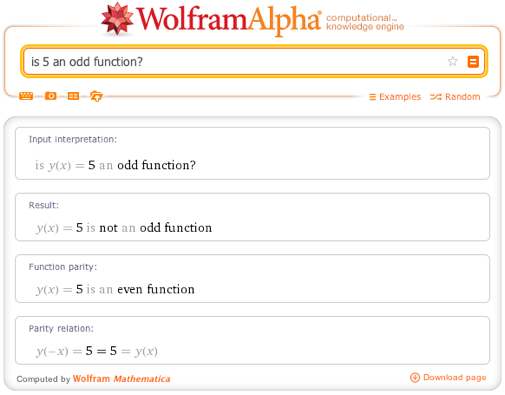An example of an odd function is sin(x):Notice that Wolfram|Alpha also tells you other important properties of sin(x), in this case the domain, range, and periodicity.

The broader math term for even- or odd-ness is parity, which is a kind of symmetry. For functions, parity refers to symmetry about the axis of the dependent variable. Functions of several variables can also have a parity, if by reversing all the independent variables you always get the same value (in the case of even parity) or the negative value (in the case of odd parity) of the dependent variable.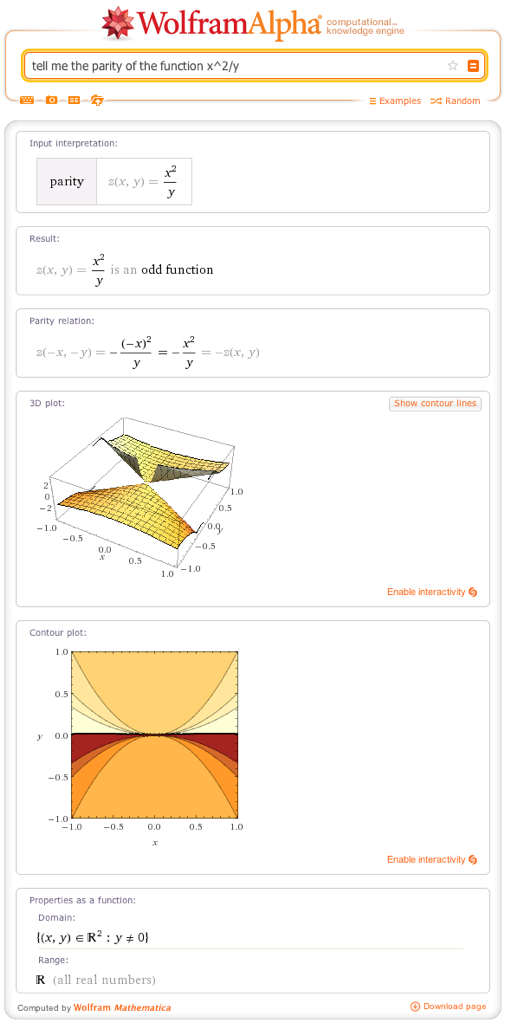Notice that both x and y were negated to find the parity. Wolfram|Alpha uses all the information and knowledge available to it to determine which of the symbols in the expression are variables. If you specify what the independent variables are by adding arguments to the dependent variable, you can change Wolfram|Alpha’s interpretation. For example, suppose you intended y to be a constant. You can indicate this by asking: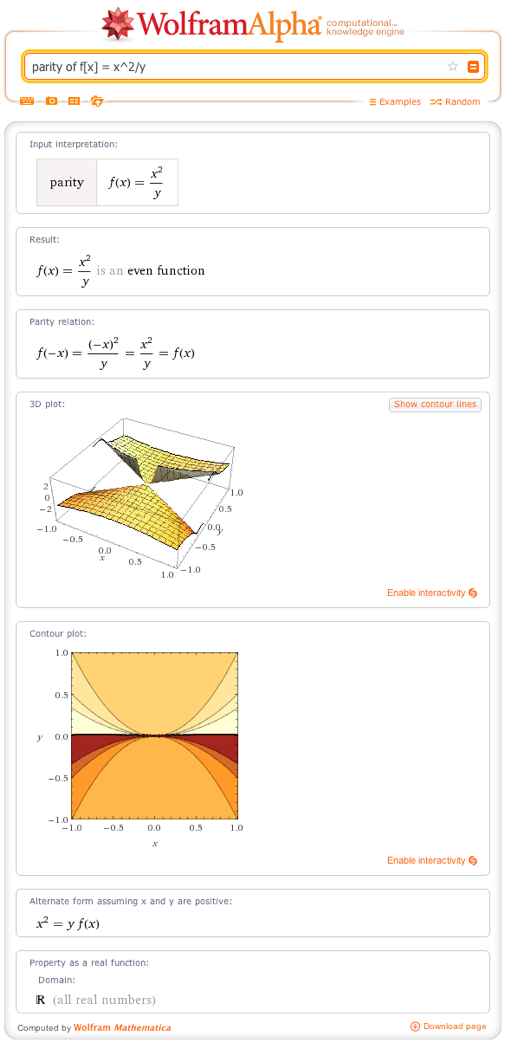Parity is one of several important symmetries in physics. It is the P in CPT-symmetry, which is the idea that for any physical event, if you could replace all the matter involved with antimatter (reversing the charge), play the event backward (reversing time), and look through a mirror, then what you would see would also be a valid physical event.

A simpler example of parity arises in undergraduate quantum mechanics, where one of the first problems is usually to find the wave function of a particle (e.g., an electron) in an impenetrable, one-dimensional box of length a, centered at the origin. Using the Schrödinger wave equation, you find two classes of solutions: sqrt(2/a) cos((2k – 1)pi x/a) and sqrt(2/a) sin(2pi k x/a), both for any integer k. The first class of solutions are even:While the second class has odd parity: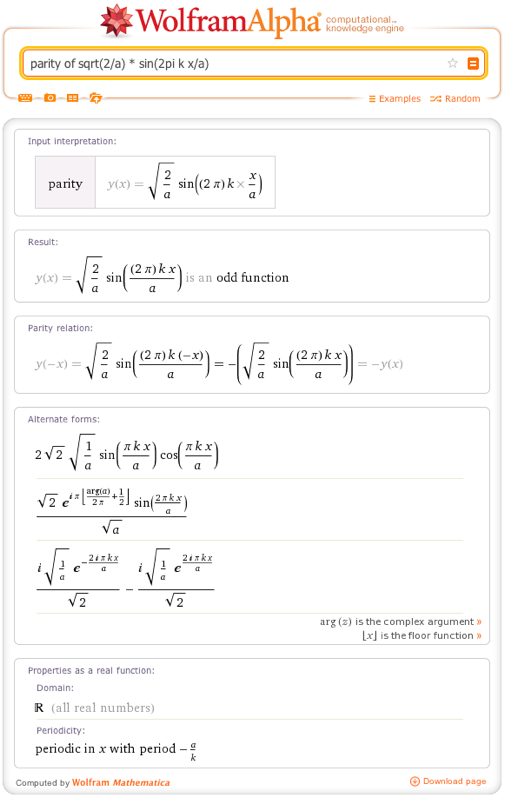In both of these cases, Wolfram|Alpha correctly interprets a as a constant, based on the presence of x. (Adding “f(x, a) =” would cause Wolfram|Alpha to interpret both as variables.)

1 Comment